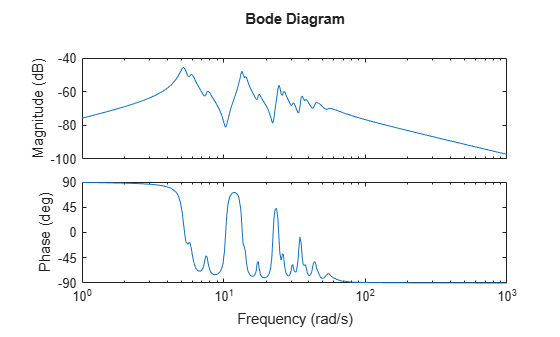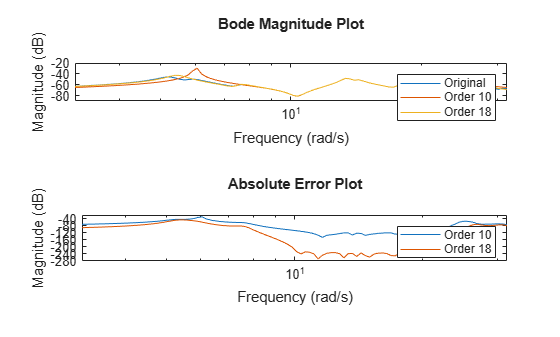# balredOptions

Create option set for model order reduction

## Syntax

```opts = balredOptions opts = balredOptions('OptionName', OptionValue) ```

## Description

`opts = balredOptions` returns the default option set for the `balred` command.

`opts = balredOptions('OptionName', OptionValue)` accepts one or more comma-separated name/value pairs. Specify `OptionName` inside single quotes.

## Input Arguments

### Name-Value Pair Arguments

`'FreqIntervals'`

Frequency intervals for computing frequency-limited Hankel singular values, specified as a matrix with two columns. Each row specifies a frequency interval `[fmin fmax]`, where `fmin` and `fmax` are nonnegative frequencies, expressed in the frequency unit of the model. When identifying low-energy states to truncate, the software computes state contributions to system behavior in these frequency ranges only. For example:

• To restrict the computation to the range between 3 rad/s and 15 rad/s, assuming the frequency unit of the model is rad/s, set `FreqIntervals` to `[3 15]`.

• To restrict the computation to two frequency intervals, 3-15 rad/s and 40-60 rad/s, use `[3 15; 40 60]`.

• To specify all frequencies below a cutoff frequency `fcut`, use `[0 fcut]`.

• To specify all frequencies above the cutoff, use ```[fcut Inf]``` in continuous time, or `[fcut pi/Ts]` in discrete time, where `Ts` is the sample time of the model.

The default value, `[]`, imposes no frequency limitation and is equivalent to `[0 Inf]` in continuous time or `[0 pi/Ts]` in discrete time. However, if you specify a `TimeIntervals` value other than `[]`, then this limit overrides `FreqIntervals = []`. If you specify both a `TimeIntervals` value and a `FreqIntervals` value, then the computation uses the union of these intervals.

If `StateElimMethod = 'MatchDC'` (the default value), then `balred` attempts to match the DC gain of the original and reduced models, even if the specified frequency intervals exclude 0. This behavior might reduce the quality of the match in the specified intervals. To improve the match within frequency intervals that exclude 0, set ```StateElimMethod = 'Truncate'```.

If both the frequency and time intervals do include DC, you can still set `StateElimMethod = 'Truncate'` to improve the match at other frequencies and times.

Default: `[]`

`'TimeIntervals'`

Time intervals for computing time-limited Hankel singular values, specified as a matrix with two columns. Each row specifies a time interval `[tmin tmax]`, where `tmin` and `tmax` are nonnegative times, expressed in the time unit of the model. When identifying low-energy states to truncate, the software computes state contributions to the system’s impulse response in these time intervals only. For example:

• To restrict the computation to the range between 3 s and 15 s, assuming the time unit of the model is seconds, set `TimeIntervals` to ```[3 15]```.

• To restrict the computation to two time intervals, 3-15 s and 40-60 s, use `[3 15; 40 60]`.

• To specify all times from zero up to a cutoff time `tcut`, use `[0 tcut]`. To specify all times after the cutoff, use `[tcut Inf]`.

The default value, `[]`, imposes no time limitation and is equivalent to `[0 Inf]`. However, if you specify a `FreqIntervals` value other than `[]`, then this limit overrides `Timeintervals = []`. If you specify both a `TimeIntervals` value and a `FreqIntervals` value, then the computation uses the union of these intervals.

If `StateElimMethod = 'MatchDC'` (the default value), then `balred` attempts to match the DC gain of the original and reduced models, even if the specified time intervals exclude `Inf`. This behavior might reduce the quality of the match in the specified intervals. To improve the match within time intervals that exclude `Inf`, set `StateElimMethod = 'Truncate'`.

If both the frequency and time intervals do include DC, you can still set `StateElimMethod = 'Truncate'` to improve the match at other frequencies and times.

Default: `[]`

`'StateElimMethod'`

State elimination method. Specifies how to eliminate the weakly coupled states (states with smallest Hankel singular values). Specified as one of the following values:

 `'MatchDC'` Discards the specified states and alters the remaining states to preserve the DC gain. `'Truncate'` Discards the specified states without altering the remaining states. This method tends to product a better approximation in the frequency domain, but the DC gains are not guaranteed to match.

Default: `'MatchDC'`

`'AbsTol, RelTol'`

Absolute and relative error tolerance for stable/unstable decomposition. Positive scalar values. For an input model G with unstable poles, `balred` first extracts the stable dynamics by computing the stable/unstable decomposition G → GS + GU. The `AbsTol` and `RelTol` tolerances control the accuracy of this decomposition by ensuring that the frequency responses of G and GS + GU differ by no more than `AbsTol` + `RelTol`*abs(G). Increasing these tolerances helps separate nearby stable and unstable modes at the expense of accuracy. See `stabsep` for more information.

Default: `AbsTol = 0; RelTol = 1e-8`

`'Offset'`

Offset for the stable/unstable boundary. Positive scalar value. In the stable/unstable decomposition, the stable term includes only poles satisfying

• `Re(s) < -Offset * max(1,|Im(s)|)` (Continuous time)

• `|z| < 1 - Offset` (Discrete time)

Increase the value of `Offset` to treat poles close to the stability boundary as unstable.

Default: `1e-8`

For additional information on the options and how to use them, see the `balred` reference page.

## Examples

collapse all

Compute a reduced-order approximation of the system given by:

`$G\left(s\right)=\frac{\left(s+0.5\right)\left(s+1.1\right)\left(s+2.9\right)}{\left(s+{10}^{-6}\right)\left(s+1\right)\left(s+2\right)\left(s+3\right)}.$`

Create the model.

`sys = zpk([-.5 -1.1 -2.9],[-1e-6 -2 -1 -3],1);`

Exclude the pole at $s=1{0}^{-6}$ from the stable term of the stable/unstable decomposition. To do so, set the `Offset` option of `balredOptions` to a value larger than the pole you want to exclude.

`opt = balredOptions('Offset',.001,'StateElimMethod','Truncate');`

Compute a second-order approximation.

`rsys = balred(sys,2,opt);`

Compare the responses of the original and reduced-order models.

`bodeplot(sys,rsys,'r--')`Reduce a high-order model with a focus on the dynamics in a particular frequency range.

Load a model and examine its frequency response.

```load('highOrderModel.mat','G') bodeplot(G)````G` is a 48th-order model with several large peak regions around 5.2 rad/s, 13.5 rad/s, and 24.5 rad/s, and smaller peaks scattered across many frequencies. Suppose that for your application you are only interested in the dynamics near the second large peak, between 10 rad/s and 22 rad/s. Focus the model reduction on the region of interest to obtain a good match with a low-order approximation. Use `balredOptions` to specify the frequency interval for `balred`.

```bopt = balredOptions('StateElimMethod','Truncate','FreqIntervals',[10,22]); GLim10 = balred(G,10,bopt); GLim18 = balred(G,18,bopt);```

Examine the frequency responses of the reduced-order models. Also, examine the difference between those responses and the original response (the absolute error).

```subplot(2,1,1); bodemag(G,GLim10,GLim18,logspace(0.5,1.5,100)); title('Bode Magnitude Plot') legend('Original','Order 10','Order 18'); subplot(2,1,2); bodemag(G-GLim10,G-GLim18,logspace(0.5,1.5,100)); title('Absolute Error Plot') legend('Order 10','Order 18');```With the frequency-limited energy computation, even the 10th-order approximation is quite good in the region of interest.

## Compatibility Considerations

expand all

Behavior changed in R2017b